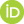Philosophy of Quantum Probability - An empiricist study of its formalism and logic

Hermens, Ronnie (2016) Philosophy of Quantum Probability - An empiricist study of its formalism and logic. UNSPECIFIED.PDF Hermens_-_PhilQProb_-_Philsci.pdf - Accepted Version Available under License Creative Commons Attribution Non-commercial No Derivatives. Download (11MB)

Abstract

The use of probability theory is widespread in our daily life (gambling, investments, etc.) as well as in scientific theories (genetics, statistical thermodynamics). In virtually all cases, calculations can be carried out within the framework of classical probability theory. A special exception is given by quantum mechanics (the physical theory that describes matter on the atomic scale), which gives rise to a new probability theory: quantum probability theory. This dissertation deals with the question of how this formalism can be understood from a philosophical and physical perspective.

The dissertation is divided into three parts. In the first part, the formalism of quantum probability theory and its relation to quantum mechanics is presented. The second part considers the possibility of reformulating quantum probability theory to embed it within classical probability. Mathematically, this possibility overlaps with the possibility of the existence of hidden variables theories of quantum mechanics: theories that attempt to solve fundamental problems in quantum mechanics by introducing additional physical properties of systems. The conclusion of this part is that, although classical reformulations of quantum probability theory are formally possible, they offer little insight into the formalism of quantum probability theory itself. The third part regards a more direct investigation of quantum probability theory. A reformulation of quantum probability theory is obtained by constructing a quantum logic on the basis of empirical non-probabilistic predictions of quantum mechanics. In this reformulation quantum probability functions are conditional probability functions on an algebra of propositions about measurements and measurement outcomes.

 Export/Citation: EndNote | BibTeX | Dublin Core | ASCII/Text Citation (Chicago) | HTML Citation | OpenURL
 Social Networking:

Item Type: Other
Creators:
CreatorsEmailORCIDHermens, Ronnie
Additional Information: PhD Dissertation, University of Groningen
Subjects: Specific Sciences > Probability/Statistics
Specific Sciences > Physics > Quantum Mechanics
Depositing User: Ronnie Hermens
Date Deposited: 01 Feb 2016 14:24
Item ID: 11865
Subjects: Specific Sciences > Probability/Statistics
Specific Sciences > Physics > Quantum Mechanics
Date: January 2016
URI: http://philsci-archive.pitt.edu/id/eprint/11865

Monthly Views for the past 3 yearsView Item